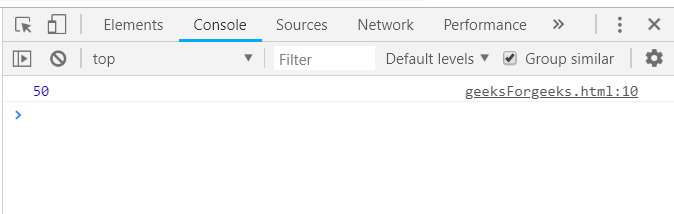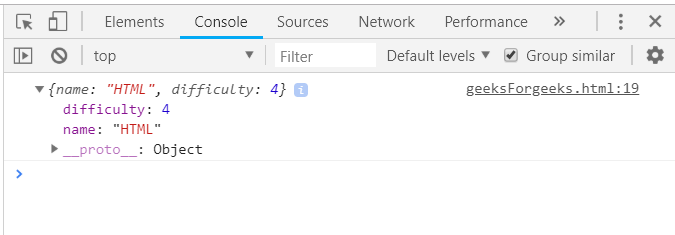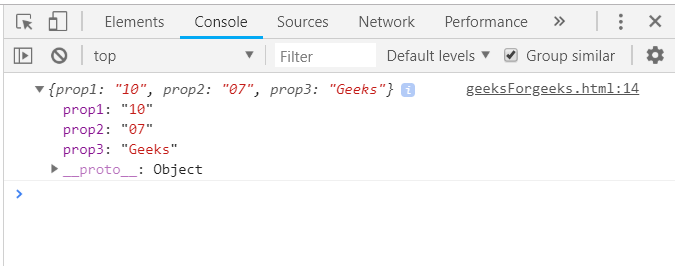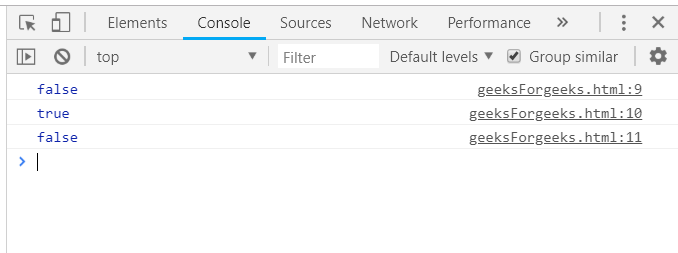# Underscore.js | min() with Examples

The Underscore.js is a JavaScript library that provides a lot of useful functions that helps in the programming in a big way like the map, filter, invoke etc even without using any built-in objects.
The _.min() function is used to find the minimum element from the list passed. If an iteratee is given, then it will be applied on each value and generate a criteria to rank the values and find the minimum element.

Syntax:

`_.min(list, [iteratee], [context])`

Parameters: This function accepts three parameters as mentioned above and described below:

• List: This parameter is used to hold the list of elements.
• Predicate: This parameter is used to hold the test condition.
• Context: This parameter is used to display the content.

Return values: The returned value is the element of the list which is minimum. A numbers list will give the least number and that of the string will give the string which is first when they are placed alphabetically.

Note: If the list is empty then ‘infinity’ will be returned.

Passing an array of numbers to the _.min function(): The ._min() function takes the element from the list one by one and do the compares the elements to find the minimum number in the list. After traversing and comparing all the elements, the _.min() function ends.

Example:

 `<``html``> ` `    ``<``head``> ` `        ``<``script` `type``=``"text/javascript"` `src``= ` `        ``"https://cdnjs.cloudflare.com/ajax/libs/underscore.js/1.9.1/underscore.js"``> ` `        `` ` `    `` ` `    ``<``body``> ` `        ``<``script` `type``=``"text/javascript"``> ` `            ``var numbers = [100, 50, 400, 66, 7900]; ` `            ``console.log(_.min(numbers)); ` `        `` ` `    `` ` `                     `

Output:Passing a list of both numbers and strings as its property to _.min() function: Passing a list of both numbers and strings and compare the elements by one of the property. Either by the numbers property or by the string property. Like here comparing on the ‘difficulty’ property. The smallest difficulty element will be returned.

Example:

 `<``html``> ` `    ``<``head``> ` `        ``<``script` `type``=``"text/javascript"` `src``= ` `        ``"https://cdnjs.cloudflare.com/ajax/libs/underscore.js/1.9.1/underscore.js"``> ` `        `` ` `    `` ` `    ``<``body``> ` `        ``<``script` `type``=``"text/javascript"``> ` `            ``var languages = [ ` `                    ``{ ` `                    ``name: 'HTML', ` `                    ``difficulty: 4 ` `                    ``}, ` `                    ``{ ` `                    ``name: 'CSS', ` `                    ``difficulty: 5 ` `                    ``} ` `                ``]; ` `            ``console.log(_.min(languages, function(o) ` `            ``{ ` `                ``return o.difficulty; ` `            ``})); ` `        `` ` `    `` ` `                     `

Output:Passing a structure of more than 1property to the _.min() function: First declare the array (here array is ‘arr’) and choose one property out of the many, on the basis of which need to find the minimum like here ‘hasLongHairs’. Console.log the variable in which this returned minimum value is stored.

Example:

 `<``html``> ` `    ``<``head``> ` `        ``<``script` `type``=``"text/javascript"` `src``= ` `        ``"https://cdnjs.cloudflare.com/ajax/libs/underscore.js/1.9.1/underscore.js"``> ` `        `` ` `    `` ` `    ``<``body``> ` `        ``<``script` `type``=``"text/javascript"``> ` `            ``var arr =  [ ` `                ``{prop1:"10", prop2:"07", prop3: "Geeks"}, ` `                ``{prop1:"12", prop2:"86", prop3: "for"}, ` `                ``{prop1:"11", prop2:"58", prop3: "Geeks."}  ` `            ``]; ` `            ``console.log(_.min(arr, function(o){return o.prop2;})); ` `        `` ` `    `` ` `                     `

Output:Passing ‘true’ and ‘false’ as the elements of the list to the _.min() function: Passing the ‘true’ and ‘false’ values to the _.min() function. The minimum of these values will be defined as ‘false’ if it is present in the list at least once otherwise answer will be ‘true’.

Example:

 `<``html``> ` `    ``<``head``> ` `        ``<``script` `type``=``"text/javascript"` `src``= ` `        ``"https://cdnjs.cloudflare.com/ajax/libs/underscore.js/1.9.1/underscore.js"``> ` `        `` ` `    `` ` `    ``<``body``> ` `        ``<``script` `type``=``"text/javascript"``> ` `           ``console.log(_.min([true, false, true])); ` `           ``console.log(_.min([true, true])); ` `           ``console.log(_.min([false, false])); ` `        `` ` `    `` ` `                     `

Output:My Personal Notes arrow_drop_upCheck out this Author's contributed articles.

If you like GeeksforGeeks and would like to contribute, you can also write an article using contribute.geeksforgeeks.org or mail your article to contribute@geeksforgeeks.org. See your article appearing on the GeeksforGeeks main page and help other Geeks.

Please Improve this article if you find anything incorrect by clicking on the "Improve Article" button below.